# Bessel F-isocrystals for reductive groups

## Abstract

We construct the Frobenius structure on a rigid connection $${{\,\mathrm{Be}\,}}_{\check{G}}$$ on $${\mathbb {G}}_{m}$$ for a split reductive group $$\check{G}$$ introduced by Frenkel–Gross. These data form a $$\check{G}$$-valued overconvergent F-isocrystal $${{\,\mathrm{Be}\,}}_{\check{G}}^{\dagger }$$ on $${\mathbb {G}}_{m,{\mathbb {F}}_p}$$, which is the p-adic companion of the Kloosterman $$\check{G}$$-local system $${{\,\mathrm{Kl}\,}}_{\check{G}}$$ constructed by Heinloth–Ngô–Yun. By studying the structure of the underlying differential equation, we calculate the monodromy group of $${{\,\mathrm{Be}\,}}_{\check{G}}^{\dagger }$$ when $$\check{G}$$ is almost simple (which recovers the calculation of monodromy group of $${{\,\mathrm{Kl}\,}}_{\check{G}}$$ due to Katz and Heinloth–Ngô–Yun), and prove a conjecture of Heinloth–Ngô–Yun on the functoriality between different Kloosterman $$\check{G}$$-local systems. We show that the Frobenius Newton polygons of $${{\,\mathrm{Kl}\,}}_{\check{G}}$$ are generically ordinary for every $$\check{G}$$ and are everywhere ordinary on $$|{\mathbb {G}}_{m,{\mathbb {F}}_p}|$$ when $$\check{G}$$ is classical or $$G_2$$.

This is a preview of subscription content, access via your institution.

1. The sum (1.1.2.1) is slightly different from the standard definition by a factor $$(-\frac{1}{\sqrt{q}})^{n-1}$$.

2. We adopt the definition of , which is different from that of  by a Tate twist.

## References

1. Abe, T.: Explicit calculation of Frobenius isomorphisms and Poincaré duality in the theory of arithmetic $$\mathscr {D}$$-modules. Rend. Semin. Mat. Univ. Padova 131, 89–149 (2014)

2. Abe, T.: Langlands program for $$p$$-adic coefficients and the petits camarades conjecture. J. Reine Angew. Math. 734, 59–69 (2018)

3. Abe, T.: Langlands correspondence for isocrystals and existence of crystalline companion for curves. J. Am. Math. Soc. 31, 921–1057 (2018)

4. Abe, T.: Around the nearby cycle functor for arithmetic $$\mathscr {D}$$-modules. Nagoya Math. J. 236, 1–28 (2019)

5. Abe, T., Caro, D.: On Beilinson’s equivalence for $$p$$-adic cohomology. Sel. Math. New Ser. 24, 591–608 (2018)

6. Abe, T., Caro, D.: Theory of weights in $$p$$-adic cohomology. Am. J. Math. 140(4), 879–975 (2018)

7. Abe, T., Esnault, H.: A Lefschetz theorem for overconvergent isocrystals with Frobenius structure. Ann. Sci. Éc. Norm. Supér. 52(4), 1243–1264 (2019)

8. Abe, T., Marmora, A.: Product formula for p-adic epsilon factors. J. Inst. Math. Jussieu 14(2), 275–377 (2015)

9. Adolphson, A., Sperber, S.: Exponential sums and Newton polyhedra: cohomology and estimates. Ann. Math. 130, 367–406 (1989)

10. André, Y.: Représentations galoisiennes et opérateurs de Bessel $$p$$-adiques. Ann. Inst. Fourier (Grenoble) 52(3), 779–808 (2002)

11. André, Y.: Filtrations de type Hasse-Arf et monodromie $$p$$-adique. Invent. Math. 148, 285–317 (2002)

12. André, Y., Baldassarri, F.: De Rham Cohomology of Differential Modules on Algebraic Varieties. Progress in Mathematics 189, Birkhäuser, Basel (2001)

13. Baumann, P., Riche, S.: Notes on the geometric Satake equivalence. In: Relative Aspects in Representation Theory, Langlands Functoriality and Automorphic Forms (CIRM Jean-Morlet Chair, Spring 2016), Lecture Notes in Mathematics, vol. 2221, pp. 1–134. Springer, Cham (2018)

14. Baldassarri, F.: Differential modules and singular points of $$p$$-adic differential equations. Adv. Math. 44(2), 155–179 (1982)

15. Baldassarri, F., Berthelot, P.: On Dwork cohomology for singular hypersurfaces. In: Adolphson, A., Baldassarri, F., Berthelot, P., Katz, N., Loeser, F. (eds.) Geometric Aspects of Dwork Theory, vol. I, pp. 177–244. De Gruyter, Berlin (2004)

16. Beilinson, A., Bernstein, J., Deligne, P., Gabber, O.: Faisceaux pervers. Astérisque 100(1982)

17. Beilinson, A., Drinfeld, V.: Quantization of Hitchin’s integrable system and Hecke eigensheaves. www.math.uchicago.edu/~mitya/langlands

18. Berthelot, P.: Cohomologie rigide et théorie de Dwork: le cas des sommes exponentielles, in $$P$$-adic cohomology. Astérisque No. 119–120(3), 17–49 (1984)

19. Berthelot, P.: Cohomologie rigide et cohomologie ridige à supports propres, première partie. Preprint (1996)

20. Berthelot, P.: $$\mathscr {D}$$-modules arithmétiques. I. Opérateurs différentiels de niveau fini. Ann. Sci. École Norm. Sup. 29(2), 18–272 (1996)

21. Berthelot, P.: Introduction à la théorie arithmétique des $$\mathscr {D}$$-modules. In: Berthelot, P., Fontaine, J.-M., Illusie, L., Kato, K., Rapoport, M. (eds.) Cohomologies $$p$$-adiques et applications arithmétiques II. Astérique, vol. 279, pp. 1–80 (2002)

22. Braden, T.: Hyperbolic localization of intersection cohomology. Transf. Groups 8(3), 209–216 (2003)

23. Braverman, A., Gaitsgory, D.: Geometric Eisenstein series. Invent. Math. 150, 287–384 (2002)

24. Carlitz, L.: A note on exponential sums. Pac. J. Math. 30, 35–37 (1969)

25. Caro, D.: $$\mathscr {D}$$-modules arithmétiques surholonomes. Ann. Sci. Éc. Norm. Supér. 42(1), 141–192 (2009)

26. Caro, D., Tsuzuki, N.: Overholonomicity of overconvergent $$F$$-isocrystals over smooth varieties. Ann. Math. 176(2), 747–813 (2012)

27. Cass, R.: Perverse $${\mathbb{F}}_p$$ sheaves on the affine Grassmannian. Preprint arXiv:1910.03377 (2019)

28. Chiarellotto, B., Tsuzuki, N.: Logarithmic growth and Frobenius filtrations for solutions of $$p$$-adic differential equations. J. Inst. Math. Jussieu 8(3), 465–505 (2009)

29. Christol, G., Mebkhout, Z.: Sur le théorème de l’indice des équations différentielles p-adiques III. Ann. Math. 151, 385–457 (2000)

30. Crew, R.: Specialization of crystalline cohomology. Duke Math. J. 53(3), 749–757 (1986)

31. Crew, R.: $$F$$-isocrystals and their monodromy groups. Ann. Sci. École Norm. Sup. 25(4), 429–464 (1992)

32. D’Addezio, M.: The monodromy groups of lisse sheaves and overconvergent F-isocrystals. Sel. Math. (N.S.) 26(45), 1–41 (2020)

33. Deligne, P.: Applications de la formule des traces aux sommes trigonométriques, In: Cohomologie Étale (SGA $$4\frac{1}{2}$$), Lecture Notes in Mathematics, 569 (1977)

34. Deligne, P.: La conjecture de Weil. II. Publ. Math. Inst. Hautes Études Sci. No. 52, pp. 137–252 (1980)

35. Deligne, P.: Catégories tannakiennes. In: The Grothendieck Festschrift. Modern Birkhäuser Classics. Birkhäuser, Boston (2007)

36. Deligne, P., Milne, J.S.: Tannakian Categories, Hodge Cycles, Motives, and Shimura Varieties. Lecture Notes in Mathematics, vol. 900. Springer, Berlin (1982)

37. Drinfeld, V., Gaitsgory, D.: On a theorem of Braden. Transform. Groups 19(2), 313–358 (2014)

38. Drinfeld, V., Kedlaya, K.: Slopes of indecomposable F-isocrystals. Pure Appl. Math. Q. 13(1), 131–192 (2017)

39. Dwork, B.: $$P$$-adic cycles. Publ. Math. Inst. Hautes Études Sci. No. 37, 27–115 (1969)

40. Dwork, B.: Bessel functions as p-adic functions of the argument. Duke Math. J. 41, 711–738 (1974)

41. Étesse, J.-Y., Le Stum, B.: Fonctions L associées aux F-isocristaux surconvergent I. Interprétation cohomologique. Math. Ann. 296, 557–576 (1993)

42. Frenkel, E., Gross, B.: A rigid irregular connection on the projective line. Ann. Math. 170, 1469–1512 (2009)

43. Fu, L., Wan, D.: L-functions for symmetric products of Kloosterman sums. J. Reine Angew. Math. 589, 79–103 (2005)

44. Gaitsgory, D.: Construction of central elements in the affine Hecke algebra via nearby cycles. Invent. Math. 144, 253–280 (2001)

45. Görtz, U., Haines, T.J.: The Jordan-Hölder series for nearby cycles on some Shimura varieties and affine flag varieties. J. Reine Angew. Math. 609, 161–213 (2007)

46. Gross, B., Reeder, M.: Arithmetic invariants of discrete Langlands parameters. Duke Math. J. 154, 431–508 (2010)

47. Heinloth, J., Ngô, B.-C., Yun, Z.: Kloosterman sheaves for reductive groups. Ann. Math. 177(1), 241–310 (2013)

48. Huyghe, C.: $$\mathscr {D}^{\dagger }(\infty )$$-affinité des schémas projectifs. Ann. Inst. Fourier (Grenoble) 48(4), 913–956 (1998)

49. Katz, N.: On the calculation of some differential Galois groups. Invent. Math. 87, 13–61 (1987)

50. Katz, N., Sums, Gauss: Kloosterman Sums, and Monodromy Groups. Annals in Mathematics Studies 116, Princeton University Press, Princeton, NJ (1988)

51. Katz, N.: Exponential Sums and Differential Equations. Annals in Mathematics Studies 124, Princeton University Press, Princeton, NJ (1990)

52. Katz, N.: From Clausen to Carlitz: low-dimensional spin groups and identities among character sums. Mosc. Math. J. 9(1), 57–89 (2009)

53. Kedlaya, K.: Semistable reduction for overconvergent F-isocrystals. I. Unipotence and logarithmic extensions. Compos. Math. 143(5), 1164–1212 (2007)

54. Kedlaya, K.: p-Adic Differential Equations. Cambridge Studies in Advanced Mathematics, 125, Cambridge University Press, Cambridge (2010)

55. Kedlaya, K.: Semistable reduction for overconvergent F-isocrystals, IV: local semistable reduction at nonmonomial valuations. Compos. Math. 147, 467–523 (2011)

56. Kedlaya, K.: Notes on isocrystals. Preprint (2016)

57. Lafforgue, V.: Estimées pour les valuations $$p$$-adiques des valeurs propres des opérateurs de Hecke. Bull. Soc. Math. Fr. 139(4), 455–477 (2011)

58. Lam, T., Templier, N.: The mirror conjecture for minuscule flag varieties, preprint, arXiv:1705.00758 (2017)

59. Liu, R., Zhu, X.: Rigidity and a Riemann–Hilbert correspondence for p-adic local systems. Invent. Math. 207, 291–343 (2017)

60. Matsuda, S.: Katz correspondence for quasi-unipotent overconvergent isocrystals. Compos. Math. 134(1), 1–34 (2002)

61. Miyatani, K.: P-adic generalized hypergeometric functions from the viewpoint of arithmetic D-modules. Am. J. Math. 142(4), 1017–1050 (2020)

62. Mirković, I., Vilonen, K.: Geometric Langlands duality and representations of algebraic groups over commutative rings. Ann. Math. 166, 95–143 (2007)

63. Ngô, B.C., Polo, P.: Résolutions de Demazure affines et formule de Casselman–Shalika géométrique. J. Algebr. Geom. 10(3), 515–547 (2001)

64. Ogus, A.: F-isocrystals and de Rham cohomology. II. Convergent isocrystals. Duke Math. J. 51(4), 765–850 (1984)

65. Ohkubo, S.: Logarithmic growth filtrations for $$(\varphi,\nabla )$$-modules over the bounded Robba ring. Compos. Math. 157(6), 1265–1301 (2021)

66. Pech, C., Rietsch, K., Williams, L.: On Landau-Ginzburg models for quadrics and flat sections of Dubrovin connections. Adv. Math. 300, 275–319 (2016)

67. Richarz, T.: A new approach to the geometric Satake equivalence. Doc. Math. 19, 209–246 (2014)

68. Richarz, T., Scholbach, J.: The motivic Satake equivalence. Math. Ann. 380, 1595–1653 (2021)

69. Richarz, T., Zhu, X.: Appendix to 

70. Shiho, A.: Crystalline fundamental groups. II. Log convergent cohomology and rigid cohomology. J. Math. Sci. Univ. Tokyo 9(1), 1–163 (2002)

71. Sperber, S.: $$p$$-adic hypergeometric functions and their cohomology. Duke Math. J. 44(3), 535–589 (1977)

72. Sperber, S.: Congruence properties of the hyper-Kloosterman sum. Compos. Math. 40(1), 3–33 (1980)

73. Tsuzuki, N.: The local index and the Swan conductor. Compos. Math. 111, 245–288 (1998)

74. Tsuzuki, N.: Minimal slope conjecture of $$F$$-isocrystals. Preprint arXiv:1910.03871 (2019)

75. Fresnel, J., van der Put, M.: Rigid Analytic Geometry and Its Applications. Progress in Mathematics, 218, Birkhäuser Boston, Inc., Boston (2004)

76. Wan, D.: Newton polygons of zeta functions and L-functions. Ann. Math. 137, 247–293 (1993)

77. Wan, D.: Variation of p-adic Newton polygons for L-functions of exponential sums. Asian J. Math. 8(3), 427–472 (2004)

78. Zhu, X.: The geometric Satake correspondence for ramified groups. Ann. Sci. Éc. Norm. Supér. 48, 409–451 (2015)

79. Zhu, X.: An introduction to affine Grassmannians and the geometric Satake equivalence. IAS/Park City Math. Ser. 24, 59–154 (2017)

80. Zhu, X.: Frenkel–Gross’ irregular connection and Heinloth–Ngô–Yun’s are the same. Sel. Math. (N.S.) 23(1), 245–274 (2017)

81. Zhu, X.: Geometric Satake, categorical traces, and arithmetic of Shimura varieties. Curr. Dev. Math. 2016, 145–206 (2016)

## Acknowledgements

We would like to thank Benedict Gross, Shun Ohkubo, Daqing Wan, Liang Xiao and Zhiwei Yun for valuable discussions. We are also grateful to an anonymous referee for his/her careful reading and valuable comments. X. Z. is partially supported by the National Science Foundation under agreement Nos. DMS-1902239 and a Simons Fellowship.

## Author information

Authors

### Corresponding author

Correspondence to Xinwen Zhu.

## Appendix A. A 2-adic proof of Carlitz’s identity and its generalization

### Appendix A. A 2-adic proof of Carlitz’s identity and its generalization

As mentioned in introduction, Carlitz  proved the following identity between Kloosterman sums:

\begin{aligned} {{\,\mathrm{Kl}\,}}(3;a)={{\,\mathrm{Kl}\,}}(2;a)^2-1,\qquad \forall ~ a\in {\mathbb {F}}_{2^s}^{\times }. \end{aligned}

In this appendix, we reprove and generalize this identity by establishing an isomorphism between two Bessel F-isocrystals $${{\,\mathrm{Be}\,}}_{2n+1}^{\dagger }$$ and $${{\,\mathrm{Be}\,}}_{{{\,\mathrm{SO}\,}}_{2n+1},{{\,\mathrm{Std}\,}}}^{\dagger }$$. The following is a restatement of Proposition 5.2.10(ii).

### Proposition A1

There exists an isomorphism between following two overcovergent F-isocrystals on $${\mathbb {G}}_{m,{\mathbb {F}}_2}$$: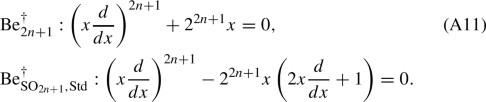Our strategy is first to show that their maximal slope quotient convergent F-isocrystals are isomorphic. Then we conclude the proposition by a dual version of a minimal slope conjecture (proposed by Kedlaya  and recently proved by Tsuzuki ) that we briefly recall in the following.

Let X be a smooth k-scheme and $$\mathscr {M}^{\dagger }$$ an overconvergent F-isocrystal on X/K. We denote the associated convergent F-isocrystal on X/K by $$\mathscr {M}$$. When the (Frobenius) Newton polygons of $$\mathscr {M}$$ are constant on X, $$\mathscr {M}$$ admits a (dual) slope filtration, that is a decreasing filtration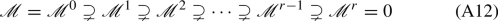of convergent F-isocrystals on X/K such that

• $$\mathscr {M}^{i}/\mathscr {M}^{i+1}$$ is isoclinic of slope $$s^i$$ and

• $$s^0>s^1>\cdots >s^{r-1}$$.

### Theorem A2

(Tsuzuki,  theorem 1.3) Let X be a smooth connected curve over k. Let $$\mathscr {M}^{\dagger },\mathscr {N}^{\dagger }$$ be two irreducible overconvergent F-isocrystals such that the corresponding convergent F-isocrystals $$\mathscr {M},\mathscr {N}$$ admit the slope filtrations $$\{\mathscr {M}^i\}$$, $$\{\mathscr {N}^i\}$$ respectively. Suppose there exists an isomorphism $$h:\mathscr {N}/\mathscr {N}^1\xrightarrow {\sim } \mathscr {M}/\mathscr {M}^1$$ of convergent F-isocrystals between the maximal slope quotients. Then there exists a unique isomorphism $$g^{\dagger }:\mathscr {N}^{\dagger }\xrightarrow {\sim } \mathscr {M}^{\dagger }$$ of overconvergent F-isocrystals, which is compatible with h as morphisms of convergent F-isocrystals.

A3 Following Dwork’s strategy [39, § 1-3], we study the maximal slope quotients of $${{\,\mathrm{Be}\,}}_{2n+1}^{\dagger }$$ and of $${{\,\mathrm{Be}\,}}_{{{\,\mathrm{SO}\,}}_{2n+1},{{\,\mathrm{Std}\,}}}^{\dagger }$$ in terms of their unique solutions at 0.

In the following, we assume $$k={\mathbb {F}}_p$$. We first recall Dwork’s congruences and show a refinement of his result in the 2-adic case. Consider for every $$i\ge 0$$, a map $$B^{(i)}(-):{\mathbb {Z}}_{\ge 0}\rightarrow K^{\times }$$ and the following congruence relation for $$0\le a<p$$ and $$n,m,s \in {\mathbb {Z}}_{\ge 0}$$:

1. (a)

$$B^{(i)}(0)$$ is a p-adic unit for all $$i\ge 0$$,

2. (b)

$$\displaystyle \frac{B^{(i)}(a+np)}{B^{(i+1)}(n)} \in R$$ for all $$i\ge 0$$,

3. (c)

$$\displaystyle \frac{B^{(i)}(a+np+mp^{s+1})}{B^{(i+1)}(n+mp^{s})} \equiv \frac{B^{(i)}(a+np)}{B^{(i+1)}(n)} \mod p^{s+1}$$ for all $$i\ge 0$$.

4. (c’)

When $$p=2$$, $$\displaystyle \frac{B^{(i)}(a+n2+m2^{s+1})}{B^{(i+1)}(n+m2^{s})} \equiv u(i,s,m) \frac{B^{(i)}(a+n2)}{B^{(i+1)}(n)} \mod 2^{s+1}$$ for all $$i\ge 0$$, where $$u(i,s,m)=1$$ if $$s\ne 1$$ and $$u(i,1,m)=1$$ or $$-1$$ depending on i and m.

If conditions (a–c) (or (a,b,c’)) are satisfied, then $$B^{(i)}(n)\in R$$ for all $$i,n\ge 0$$. We set

\begin{aligned}&F^{(i)}(x)=\sum _{j=0}^{\infty } B^{(i)}(j) x^j ~ \in K \llbracket x \rrbracket , \\&F^{(i)}_{m,s}(x)=\sum _{j=mp^s}^{(m+1)p^{s}-1} B^{(i)}(j) x^j~ \in K[x],\quad s\ge 0. \end{aligned}

We write $$F^{(i)}_{0,s}$$ by $$F^{(i)}_{s}$$ for simplicity.

### Theorem A4

(i) [39, theorem 2] If conditions (a–c) are satisfied, then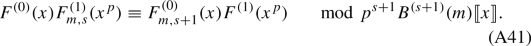(i$$^{\prime }$$) If conditions (a,b,c$$^{\prime }$$) are satisfied (in particular $$p=2$$), then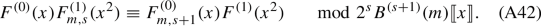(ii) [39, theorem 3] Under the assumption of (i) or (i’) and suppose moreover that

1. (d)

$$B^{(i)}(0)=1$$ for $$i\ge 0$$.

2. (e)

$$B^{(i+r)}=B^{(i)}$$ for all $$i\ge 0$$ and some fixed $$r\ge 1$$.

Let U be the open subscheme of $${\mathbb {A}}^1_{k}$$ defined by $$F_{1}^{(i)}(x)\ne 0$$, for $$i=0,1,\ldots ,r-1$$. Then the limit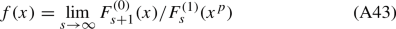defines a global function on the formal open subscheme $$\mathfrak {U}$$ of $$\widehat{{\mathbb {A}}}^{1}_R$$ associated to U, which takes p-adic unit value at each rigid point of $$\mathfrak {U}^{{{\,\mathrm{rig}\,}}}$$.

We prove assertion (i’) in the end (A11). We briefly explain Dwork’s result (ii) in the language of formal schemes. The assumption implies that $$F_s^{(i)}\ne 0$$ on U (cf.  3.4). For $$s\ge 1$$, the congruences (A41) or ((A42)) imply that

\begin{aligned} F_{s+1}^{(0)}(x)/ F_s^{(1)}(x^p)= F_s^{(0)}(x)/F_{s-1}^{(1)}(x^p) \quad \in \Gamma (\mathfrak {U},\mathscr {O}_{\mathfrak {U}}/p^{s-1}\mathscr {O}_{\mathfrak {U}}). \end{aligned}

This allows us to use (A43) to define a global function f of $$\mathscr {O}_{\mathfrak {U}}$$.

A5 Let $$F(x)=\sum _{j\ge 0} B(j) x^{j}$$ be a formal power series in $$R\llbracket x \rrbracket$$. We say F satisfies Dwork’s congruences if by setting $$B^{(i)}(j)=B(j)$$ for every $$i\ge 0$$, conditions of Theorem A4(ii) are satisfied.

We take such a function F and then we obtain a function $$f\in \Gamma (\mathfrak {U},\mathscr {O}_{\mathfrak {U}})$$ coinciding with $$F(x)/F(x^p)$$ in $$K\{x\}$$ (2.3.1.1) (i.e. the open unit disc). Moreover, by [39, lemma 3.4(ii)], there exists a function $$\eta \in \Gamma (\mathfrak {U},\mathscr {O}_{\mathfrak {U}})$$ coinciding with $$F'(x)/F(x)$$ in $$K\{x\}$$ defined by

\begin{aligned} \eta (x)\equiv F'_{s+1}(x)/F_{s+1}(x) \mod p^{s}. \end{aligned}

The functions f(x) and $$\eta (x)$$ satisfy a differential equation:

\begin{aligned} \frac{f'(x)}{f(x)}+px^{p-1}\eta (x^{p})=\eta (x). \end{aligned}

Note that $$f(0)=F(0)/F(0)=1$$. Then we deduce that the following corollary.

### Corollary A6

The connection $$d- \eta$$ on the trivial bundle $$\mathscr {O}_{\mathfrak {U}^{{{\,\mathrm{rig}\,}}}}$$ and the function f form a unit-root convergent F-isocrystal $$\mathscr {E}_F$$ on U/K, whose Frobenius eigenvalue at 0 is 1.

A7. Let $$\mathscr {M}^{\dagger }$$ be an overconvergent F-isocrystal on $${\mathbb {G}}_{m,k}$$ over K of rank r whose underlying bundle is trivial and the connection is defined by a differential equation:

\begin{aligned} P(\delta )=\delta ^r+p_{r}\delta ^{r-1}+\cdots + p_{1}=0, \end{aligned}

where $$\delta =x\frac{d}{dx}$$, $$p_i\in \Gamma (\widehat{{\mathbb {A}}}_R^1,\mathscr {O}_{\widehat{{\mathbb {A}}}_R^1})[\frac{1}{p}]$$. We assume moreover that $$\mathscr {M}^{\dagger }$$ is unipotent at 0 with a maximal unipotent local monodromy. Then $$\mathscr {M}^{\dagger }$$ extends to a log convergent F-isocrystal $$\mathscr {M}^{\log }$$ on $$({\mathbb {A}}^1,0)$$ and its Frobenius slopes at 0 are

\begin{aligned} s^0>s^1=s^0-1>\cdots > s^{r-1}=s^0-(r-1). \end{aligned}

Note that $$\mathscr {M}^{\dagger }$$ is indecomposable in $$F\text {-}{{\,\mathrm{Isoc}\,}}^{\dagger }({\mathbb {G}}_{m,k}/K)$$ and so is $$\mathscr {M}$$ in $$F\text {-}{{\,\mathrm{Isoc}\,}}({\mathbb {G}}_{m,k}/K)$$. Then by Drinfeld–Kedlaya’s theorem on the generic Frobenius slopes , we deduce property (i):

(i) The generic Frobenius slopes (mult-)set is $$\{s^0,\ldots ,s^{r-1}\}$$ with $$s^i=s^0-(i-1)$$.

(ii) In view of (2.3.1.2), the differential equation $$D=0$$ admits a unique solution at 0:

\begin{aligned} F(x)=\sum _{n\ge 0} A(n) x^n \quad \in K\{x\},\quad \text { with A(0)=1}. \end{aligned}

### Proposition A8

Suppose the function F(x) satisfies Dwork’s congruences (A5) and let $$\mathscr {E}_F$$ be the associated unit-root convergent F-isocrystal on $$U \subset {\mathbb {A}}^1_k$$. Then

(i) There exists an epimorphism of log convergent isocrystals $$\mathscr {M}^{\log }\rightarrow \mathscr {E}_F$$ on (U, 0).

(ii) As convergent isocrystals, $$\mathscr {E}_F$$ coincides with the maximal slope quotient $$\mathscr {M}^{\log }/\mathscr {M}^{\log ,1}$$ of $$\mathscr {M}^{\log }$$ (A12).

### Proof

(i) We set $$A=\Gamma (\mathfrak {U},\mathscr {O}_{\mathfrak {U}})[\frac{1}{p}]$$. We claim that there exists a decomposition of differential operators: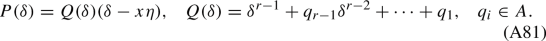Indeed, by the Euclidean algorithm [54, 5.5.2], there exists $$r\in A$$ such that $$P=Q(\delta -x\eta )+r$$. By evaluating the above identity at F (in the ring $$K\{x\}$$ containing A), we obtain

\begin{aligned} P(\delta )(F)=0=Q(\delta )(\delta -x\eta )(F)+rF=rF. \end{aligned}

Then we deduce $$r=0$$ and (A81) follows.

Let $$e_1,\ldots ,e_r$$ be a basis of $$\mathscr {M}$$ such that $$\nabla _{\delta }(e_i)=e_{i+1}, 1\le i\le r-1$$ and $$\nabla _{\delta }(e_r)=-(p_r e_r+\cdots +p_1 e_1)$$. We consider a free $$\mathscr {O}_{\mathfrak {U}^{{{\,\mathrm{rig}\,}}}}$$-module with a log connection $$\mathscr {N}$$ with a basis $$f_1,\ldots ,f_{r-1}$$ and the connection defined by $$\nabla _{\delta }(f_i)=f_{i+1}$$, $$\nabla _{\delta }(f_{r-1})=-(q_{r-1} f_{r-1}+\cdots +q_{1}f_{1})$$. By (A81), the morphism $$f_1\mapsto e_2- x\eta e_1$$ induces a horizontal monomorphism $$\mathscr {N}\rightarrow \mathscr {M}^{\log }$$ whose cokernel is isomorphic to $$\mathscr {E}_F$$.

(ii) Note that $${{\,\mathrm{Pic}\,}}(\mathfrak {U}^{{{\,\mathrm{rig}\,}}})\simeq {{\,\mathrm{Pic}\,}}(U)$$ [75, 3.7.4] is trivial. Then the rank one convergent isocrystal $$\mathscr {M}^{\log }/\mathscr {M}^{\log ,1}$$ can be represented as a connection $$d-\lambda$$ on the trivial bundle $$\mathscr {O}_{\mathfrak {U}^{{{\,\mathrm{rig}\,}}}}$$.

Since $$\mathscr {M}^{\log }$$ has a maximal unipotent at 0, the rank one quotient of the restriction $$\mathscr {M}^{\log }|_0$$ of $$\mathscr {M}^{\log }$$ at the open unit disc around 0 is unique (2.3.1). In particular, $$d-\lambda$$ kills the unique solution F of $$P(\delta )=0$$. By analytic continuation, we have $$\lambda =\eta$$ and the assertion follows. $$\square$$

### Remark A9

The unique solution F(x) belongs to the ring $$K\llbracket x \rrbracket _0 = R \llbracket x \rrbracket \otimes _R K$$ of bounded functions on open unit disc, which is a subring of $$K\{x\}$$. Assertion (ii) can be viewed as an example of Dwork–Chiarellotto–Tsuzuki conjecture on the comparison between the log-growth filtration (of solutions) and Frobenius slope filtration . This conjecture was recently proved by Ohkubo .

### Proof of Proposition A1

We set $$k={\mathbb {F}}_2$$ and apply the above discussions to overconvergent F-isocrystals $$\mathscr {M}^{\dagger }={{\,\mathrm{Be}\,}}_{2n+1}^{\dagger }$$ and $$\mathscr {N}^{\dagger }={{\,\mathrm{Be}\,}}_{{{\,\mathrm{SO}\,}}_{2n+1},{{\,\mathrm{Std}\,}}}^{\dagger }$$ on $${\mathbb {G}}_{m,{\mathbb {F}}_2}/K$$ (A11). Their unique solutions at 0 are:

\begin{aligned} F(x)= \sum _{r\ge 0} \frac{(-2)^{(2n+1)r}}{(r!)^{2n+1}} x^r,\qquad G(x)=\sum _{r\ge 0} \frac{2^{(2n+1)r}(2r-1)!!}{(r!)^{2n+1}} x^r. \end{aligned}

In the following lemma, we show that F and G satisfy Dwork’s congruences and that the associated maximal slope quotients $$\mathscr {E}_F$$ and $$\mathscr {E}_G$$ (A8) are isomorphic. Then Proposition A1 follows from theorem A2 and the following lemma. $$\square$$

### Lemma A10

(i) The functions F(x) and G(x) satisfy Dwork’s congruences (A5) and define unit-root convergent F-isocrystals $$\mathscr {E}_F$$ and $$\mathscr {E}_G$$ on $${\mathbb {A}}_k^1$$ respectively.

(ii) The function F(x)/G(x) extends to a global function of $$\widehat{{\mathbb {A}}}^1_R$$ and induces an isomorphism $$\mathscr {E}_G\xrightarrow {\sim } \mathscr {E}_F$$.

### Proof

(i) Conditions (a,b,d,e) are easy to verified. The coefficients of F(x) (resp. G(x)) satisfy condition (c’) (resp. (c)), that is

\begin{aligned}&\frac{(-2)^{(2n+1)(a+\ell 2+m2^{s+1})}/((a+\ell 2+m2^{s+1})!)^{2n+1}}{(-2)^{(2n+1)(\ell +m2^{s})}/((\ell +m2^{s})!)^{2n+1}} \\&\quad \equiv u(s,m) \frac{ (-2)^{(2n+1)(a+\ell 2)}/ ((a+\ell 2)!)^{2n+1}}{(-2)^{(2n+1)\ell }/ (\ell !)^{2n+1}} \mod 2^{s+1}, \end{aligned}

where $$u(1,m)=(-1)^m$$ and $$u(s,m)=1$$ if $$s\ne 1$$, and

\begin{aligned}&\frac{(2(a+\ell 2+m2^{s+1})-1)!! 2^{(2n+1)(a+\ell 2+m2^{s+1})}/((a+\ell 2+m2^{s+1})!)^{2n+1}}{(2(\ell +m2^{s})-1)!!2^{(2n+1)(\ell +m2^{s})}/((\ell +m2^{s})!)^{2n+1}} \\&\quad \equiv \frac{(2(a+\ell 2)-1)!!2^{(2n+1)(a+\ell 2)}/ ((a+\ell 2)!)^{2n+1}}{(2\ell -1)!!2^{(2n+1)\ell }/ (\ell !)^{2n+1}} \mod 2^{s+1}. \end{aligned}

Since $$F_1(x)\equiv G_1(x)\equiv 1\mod 2$$, the F-isocrystals $$\mathscr {E}_{F},\mathscr {E}_G$$ are defined over $${\mathbb {A}}_k^1$$.

(ii) We set $$B^{(0)}(r)=\frac{(-2)^{(2n+1)r}}{(r!)^{2n+1}}$$ and $$B^{(1)}(r)=\frac{2^{(2n+1)r}(2r-1)!!}{(r!)^{2n+1}}$$ and $$B^{(i+2)}=B^{(i)}$$. Then these sequences satisfy conditions (a,b,c’,d,e). For condition (c’), the constants u(i, 1, m) are given by

\begin{aligned} u(0,1,m)=1, \quad u(1,1,m)=(-1)^m,\quad u(i+2,1,m)=u(i,1,m). \end{aligned}

Since $$F_1(x)\equiv G_1(x)\equiv 1\mod 2$$, $$F(x)/G(x^2)$$ extends to a global function of $$\mathscr {O}_{\widehat{{\mathbb {A}}}_R^1}$$ by Theorem A4 and so is F(x)/G(x). Then the assertion follows. $$\square$$

A11 Proof of Theorem A4(i’). We prove assertion (i’) by modifying the argument of [39, theorem 2]. Note that condition (c’) implies the following congruence relation: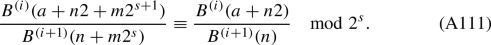When $$n<0$$, we set $$B^{(i)}(n)=0$$. We set $$A=B^{(0)}$$, $$B=B^{(1)}$$ and for $$a\in \{0,1\}$$, $$j,N\in {\mathbb {Z}}$$, we set

\begin{aligned}&U_{a}(j, N) =A(a+2(N-j)) B(j)-B(N-j) A(a+2j), \\&H_{a}(m, s, N) = \sum _{j=m2^s}^{(m+1)2^s-1} U_{a}(j, N). \end{aligned}

Then the assertion is equivalent toBy condition (b), we have $$A(a+2m)/B(m)\in R$$ and hence

\begin{aligned} U_a(m,N) \equiv 0 \mod B(m). \end{aligned}

Then equation (A112) for $$s=0$$ follows from the fact that $$H_a(m,0,N)=U_a(m,N)$$.

We now prove by induction on s. We write the induction hypothesis

\begin{aligned} \alpha _s: H_a(m,u,N)\equiv 0 \mod 2^u B^{(u+1)}(m),\quad \text {for } u\in [0,s), m,N\ge 0. \end{aligned}

We may assume $$\alpha _{s}$$ for fixed $$s\ge 1$$. The main step is to show for $$0\le t\le s$$ that

\begin{aligned}&\beta _{t,s}: v(s,t,m) H_a(m,s,N+m2^s)\\&\quad \equiv \sum _{j=0}^{2^{s-t}-1} B^{(t+1)}(j+m2^{s-t})H_a(j,t,N)/B^{(t+1)}(j)\mod 2^s B^{(s+1)}(m), \end{aligned}

where $$v(s,t,m)=1$$ or $$-1$$ depending on stm.

We list some elementary facts (cf. [39, 2.5–2.7])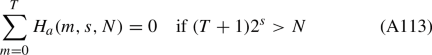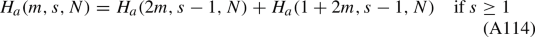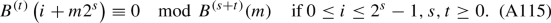We first prove $$\beta _{0,s}$$. We have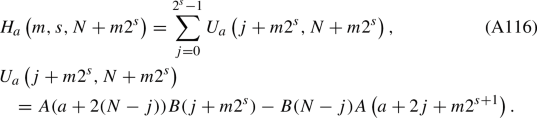By (A111), we have

\begin{aligned} A\left( a+2 j+m 2^{s+1}\right) = A(a+2j)B(j+m2^s)/B(j)+X_j 2^s B(j+m 2^s), \end{aligned}

where $$X_j\in R$$. Then the right hand side of (A116) is

\begin{aligned} B\left( j+m 2^{s}\right) \biggl (U_{a}(j, N) / B(j)-2^{s} X_{j} B(N-j)\biggr ). \end{aligned}

Since $$U_{a}(j,N)=H_a(j,0,N)$$, we obtain

\begin{aligned} H_{a}\left( m, s, N+m 2^{s}\right)= & {} \sum _{j=0}^{2^{s}-1} B\left( j+m 2^{s}\right) H_{a}(j, 0, N) / B(j)\\&-2^{s} \sum _{j=0}^{2^{s}-1} X_{j} B\left( j+m 2^{s}\right) B(N-j). \end{aligned}

Since $$X_jB(N-j)\in R$$, it follows from (A115) ($$B=B^{(1)}$$) that the second sum is congruent to zero modulo $$2^s B^{(s+1)}(m)$$. This proves $$\beta _{0,s}$$ with $$v(s,0,m)=1$$.

With s fixed, $$s\ge 1$$, t fixed, $$0\le t \le s-1$$, we show that $$\beta _{t,s}$$ together with $$\alpha _s$$ imply $$\beta _{t+1,s}$$. To do this we put $$j=\mu +2i$$ in the right side of $$\beta _{t,s}$$ and write it in the form

\begin{aligned} \sum _{\mu =0}^{1} \sum _{i=0}^{2^{s-t-1}} B^{(t+1)}\left( \mu + 2i+m 2^{s-i}\right) H_{a}(\mu +2 i, t, N) / B^{(t+1)}(\mu +2i). \end{aligned}

By condition (c’), we have,

\begin{aligned}&B^{(t+1)}\left( \mu +2i+m2^{s-t}\right) \\&\quad = u(t+1,s-t-1,m)\left( B^{(t+1)}(\mu +2 i) B^{(t+2)}\left( i+m2^{s-t-1}\right) / B^{(t+2)}(i)\right) \\&\qquad +X_{i, \mu } 2^{s-t} B^{(t+2)}\left( i+m 2^{s-t-1}\right) , \end{aligned}

where $$X_{i,\mu }\in R$$. Thus the general term in the above double sum is

\begin{aligned} u(t+1,s-t-1,m)\biggl (B^{(t+2)}(i+m2^{s-t-1})H_a(\mu +2i,t,N)/B^{(t+2)}(i)\biggr )+Y_{i,\mu }, \end{aligned}

where the error term:

\begin{aligned} Y_{i, \mu }=X_{i, \mu } 2^{s-t} B^{(t+2)}\left( i+m 2^{s-t-1}\right) H_{a}(\mu +2 i, t, N) / B^{(t+1)}(\mu +2i). \end{aligned}

For this error term, since $$t<s$$, we can apply $$\alpha _s$$ to conclude that

\begin{aligned} Y_{i,\mu }\equiv 0 \mod B^{(t+2)}(i+m2^{s-t-1})2^s. \end{aligned}

Then we can use (A115) to conclude that

\begin{aligned} Y_{i,\mu }\equiv 0 \mod 2^s B^{(s+1)}(m). \end{aligned}

After modulo $$2^s B^{(s+1)}(m)$$, the right side of $$\beta _{t,s}$$ is equal to

\begin{aligned}&u(t+1,s-t-1,m)\sum _{\mu =0}^{1} \sum _{i=0}^{2^{s-t-1}-1} B^{(t+2)}\left( i+m2^{s-t-1}\right) \\&\quad \times H_{a}(\mu +2 i, t, N) / B^{(t+2)}(i).\end{aligned}

By reversing the order of summation and using (A114), the above sum is the same as

\begin{aligned}&u(t+1,s-t-1,m)\sum _{i=0}^{2^{s-t-1}-1} B^{(t+2)}\left( i+m2^{s-t-1}\right) \\&\quad \times H_{a}(i, t+1, N) / B^{(t+2)}(i),\end{aligned}

which proves $$\beta _{t+1,s}$$. In particular, we obtain $$\beta _{s,s}$$, which states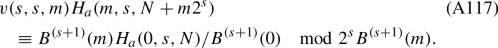We now consider the statement (with s fixed before)

\begin{aligned} \gamma _{N}:H_a(0,s,N)\equiv 0 \mod 2^s. \end{aligned}

We know that $$\gamma _N$$ is true for $$N<0$$. Let $$N'$$ (if it exists) be the minimal value of N for which $$\gamma _{N'}$$ fails. For $$m\ge 1$$, since $$B^{(s+1)}(0)$$ is a unit, we have by (A117)

\begin{aligned} H_a(m,s,N')\equiv & {} v(s,s,m) B^{(s+1)}(m)H_a(0,s,N'-m2^s)/B^{(s+1)}(0)\\\equiv & {} 0 \mod 2^s. \end{aligned}

Applying this to (A113), we obtain that

\begin{aligned} H_a(0,s,N')\equiv 0 \mod 2^s. \end{aligned}

Thus $$\gamma _N$$ is valid for all N and Eq. (A117) implies $$\alpha _{s+1}$$. This proves assertion (i$$^{\prime }$$). $$\square$$

## Rights and permissions

Reprints and Permissions